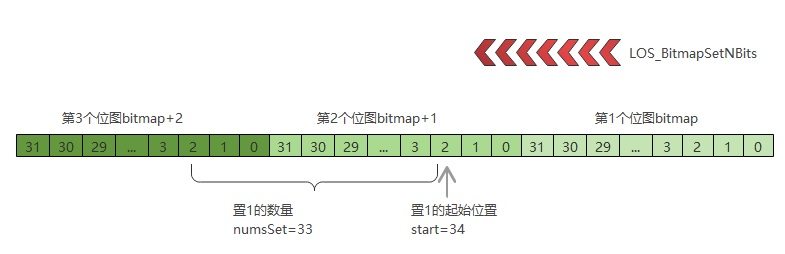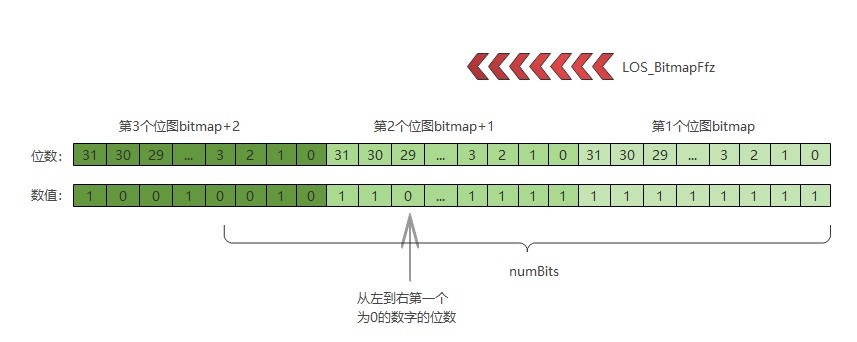zhushangyuan_

1收藏

# 鸿蒙轻内核A核源码分析系列二 数据结构-位图操作

## 1 位操作的宏定义

``````⑴  #define OS_BITMAP_MASK 0x1FU
``````

``````    #define _ONE(x) (1 + ((x) - (x)))
#define BIT(n)  (1U << (n))
#define BIT_GET(x, bit) ((x) & (_ONE(x) << (bit)))
#define BIT_SHIFT(x, bit) (((x) >> (bit)) & 1)
#define BITS_GET(x, high, low) ((x) & (((_ONE(x) << ((high) + 1)) - 1) & ~((_ONE(x) << (low)) - 1)))
#define BITS_SHIFT(x, high, low) (((x) >> (low)) & ((_ONE(x) << ((high) - (low) + 1)) - 1))
#define BIT_SET(x, bit) (((x) & (_ONE(x) << (bit))) ? 1 : 0)
#define BITMAP_BITS_PER_WORD (sizeof(UINTPTR) * 8)
#define BITMAP_NUM_WORDS(x) (((x) + BITMAP_BITS_PER_WORD - 1) / BITMAP_BITS_PER_WORD)
#define BITMAP_WORD(x) ((x) / BITMAP_BITS_PER_WORD)
#define BITMAP_BIT_IN_WORD(x) ((x) & (BITMAP_BITS_PER_WORD - 1))
#define BITMAP_FIRST_WORD_MASK(start) (~0UL << ((start) % BITMAP_BITS_PER_WORD))
(((nbits) % BITMAP_BITS_PER_WORD) ? (1UL << ((nbits) % BITMAP_BITS_PER_WORD)) - 1 : ~0UL)
#define BITMAP_BITS_PER_INT (sizeof(INTPTR) * 8)
#define BITMAP_BIT_IN_INT(x) ((x) & (BITMAP_BITS_PER_INT - 1))
#define BITMAP_INT(x) ((x) / BITMAP_BITS_PER_INT)
#define BIT_MASK(x) (((x) >= sizeof(UINTPTR) * 8) ? (0UL - 1) : ((1UL << (x)) - 1))
``````

## 2 位操作常用功能

`OpenHarmony`鸿蒙轻内核的位操作模块提供标志位的置1和清0操作，可以改变标志位的内容，同时还提供获取状态字中标志位为1的最高位和最低位的功能。用户也可以对系统的寄存器进行位操作。位操作提供了7个`API`，进行置1、清0、获取为1的最高、最低位等操作，如下：

LOS_BitmapSet 对状态字的某一标志位进行置1操作
LOS_BitmapClr 对状态字的某一标志位进行清0操作
LOS_HighBitGet 获取状态字中为1的最高位
LOS_LowBitGet 获取状态字中为1的最低位
LOS_BitmapSetNBits 对状态字的连续标志位进行置1操作
LOS_BitmapClrNBits 对状态字的连续标志位进行清0操作
LOS_BitmapFfz 获取从最低有效位开始的第一个0的bit位

### 2.1 LOS_BitmapSet()对状态字的某一标志位进行置1操作

``````VOID LOS_BitmapSet(UINT32 *bitmap, UINT16 pos)
{
if (bitmap == NULL) {
return;
}

*bitmap |= 1U << (pos & OS_BITMAP_MASK);
}
``````

### 2.2 LOS_BitmapClr()对状态字的某一标志位进行清0操作

``````VOID LOS_BitmapClr(UINT32 *bitmap, UINT16 pos)
{
if (bitmap == NULL) {
return;
}

*bitmap &= ~(1U << (pos & OS_BITMAP_MASK));
}
``````

### 2.3 LOS_HighBitGet()获取状态字中为1的最高位

``````UINT16 LOS_HighBitGet(UINT32 bitmap)
{
if (bitmap == 0) {
return LOS_INVALID_BIT_INDEX;
}

}
``````

### 2.4 LOS_LowBitGet()获取状态字中为1的最低位

``````UINT16 LOS_LowBitGet(UINT32 bitmap)
{
if (bitmap == 0) {
return LOS_INVALID_BIT_INDEX;
}

return CTZ(bitmap);
}
``````

### 2.5 LOS_BitmapSetNBits()对状态字的连续标志位进行置1操作⑸处如果条件成立，说明需要置1操作需要跨多个状态字进行操作，代码会一个状态字处理完毕，再去处理下一个状态字。⑹处把当前状态字的相应的`bit`位进行置1操作，然后执行⑺把剩余需要置1的位数减去已经置1的位数。⑻处更新`bitsToSet``maskToSet`，然后指针`p`指向下一个状态字。⑼处如果需要置1的位数大于0，并且此时已经可以在一个状态字内完成操作，执行⑽处计算需要置1操作的掩码，从`bit`开始位到结束位需要进行置1。⑾处代码执行置1操作，完成对状态字的连续标志位进行置1操作。

``````VOID LOS_BitmapSetNBits(UINTPTR *bitmap, UINT32 start, UINT32 numsSet)
{
⑴  UINTPTR *p = bitmap + BITMAP_WORD(start);
⑵  const UINT32 size = start + numsSet;
⑶  UINT16 bitsToSet = BITMAP_BITS_PER_WORD - (start % BITMAP_BITS_PER_WORD);

⑸  while (numsSet > bitsToSet) {
⑺      numsSet -= bitsToSet;
⑻      bitsToSet = BITMAP_BITS_PER_WORD;
p++;
}
⑼  if (numsSet) {
}
}
``````

### 2.6 LOS_BitmapClrNBits()对状态字的连续标志位进行清0操作

``````VOID LOS_BitmapClrNBits(UINTPTR *bitmap, UINT32 start, UINT32 numsClear)
{
UINTPTR *p = bitmap + BITMAP_WORD(start);
const UINT32 size = start + numsClear;
UINT16 bitsToClear = BITMAP_BITS_PER_WORD - (start % BITMAP_BITS_PER_WORD);

while (numsClear >= bitsToClear) {
numsClear -= bitsToClear;
bitsToClear = BITMAP_BITS_PER_WORD;
p++;
}
if (numsClear) {
}
}
``````

### 2.8 LOS_BitmapFfz()获取从最低有效位开始的第一个0的bit位

``````/* find first zero bit starting from LSB */
STATIC INLINE UINT16 Ffz(UINTPTR x)
{
return __builtin_ffsl(~x) - 1;
}
````````````INT32 LOS_BitmapFfz(UINTPTR *bitmap, UINT32 numBits)
{
INT32 bit, i;

⑴  for (i = 0; i < BITMAP_NUM_WORDS(numBits); i++) {
⑵      if (bitmap[i] == OS_BITMAP_WORD_MASK) {
continue;
}
⑶      bit = i * BITMAP_BITS_PER_WORD + Ffz(bitmap[i]);
⑷      if (bit < numBits) {
return bit;
}
return -1;
}
return -1;
}
``````

## 小结

3

2条回复

/

mb609898e2cfb86

2021-6-8 11:39:29
zhushangyuan_ 回复了 mb609898e2cfb86

2021-6-8 11:43:16帖子
视频
声望
粉丝
相关问题
社区精华内容